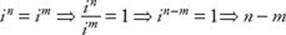﻿ ﻿Exercises - Imaginary and Complex Numbers - MISCELLANEOUS TOPICS - SAT SUBJECT TEST MATH LEVEL 1

## MISCELLANEOUS TOPICS## CHAPTER 17Imaginary and Complex Numbers### Exercises1. If a and b are real numbers and if ai 2 = bi 4, which of the following must be true?

I. a = b

II. a = –b

III. |a| = |b|

(A) I only

(B) II only

(C) III only

(D) II and III only

(E) I, II, and III

2. Which of the following is a negative number?

(A) i 25

(B) i 50

(C) i 75

(D) i 100

(E) i 200

3. Which of the following is equal to (1 + i )2?

(A) –2

(B) 0

(C) 2

(D) –2i

(E) 2i

4. If a and b are real numbers, which of the following is equal to (a + bi )(abi )?

(A) a2b2

(B) a2 + b2

(C) a2 + 2abib2

(D) a2 – 2abib2

(E) a2 – 2abi + b2

5. If a, b, c, and d are consecutive positive integers, what is the value of i a+ i b+ i c+ i d?

(A) 0

(B) –1

(C) 1

(D) i

(E) It cannot be determined from the information given.

6. If i m = i n, which of the following must be true?

I. m = n

II. m + n is even

III. nm is a multiple of 4.

(A) I only

(B) III only

(C) I and III only

(D) II and III only

(E) I, II, and III

 1. (D) 3. (E) 5. (A) 2. (B) 4. (B) 6. (D)

Solutions

Each of the problems in this set of exercises is typical of a question you could see on a Math 1 test. When you take the model tests in this book and, in particular, when you take the actual Math 1 test, if you get stuck on questions such as these, you do not have to leave them out—you can almost always answer them by using one or more of the strategies discussed in the “Tactics” chapter. The solutions given here do not depend on those strategies; they are the correct mathematical ones.

See Important Tactics for an explanation of the symbol ⇒, which is used in several answer explanations.

1. (D) Since i 2 = –1 and i 4 = 1, ai 2 = bi 4a(–1) = b(1)a = b. Hence a = –b and |a| = |–b| = |b|. (II and III are true.) Statement I could be true—if a and b were each 0—but does not have to be true: –2i 2 = 2i 4, but –22. Only statements II and III are true.

2. (B) Since 100 and 200 are multiples of 4, i 100 = i 200 = 1. The remainders when 25, 50, and 75 are divided by 4 are 1, 2, and 3, respectively. Therefore, i 25 = i, i 50 = –1, and i 75 = –i. Of these, only i 50 is a negative number.

3. (E) (1 + i )2 = (1 + i )(1 + i ) = 1 + 2i + i 2 = 1 + 2i + (–1) = 2i

4. (B) (a + bi )(abi ) = a2abi + abib2i 2 = a2b2(–1) = a2 + b2

5. (A) Since the values of the power of i repeat indefinitely in groups of four, i a, i b, i c, i d are in some order: i, –1, –i, and 1. So their sum is i + (–1) + (–i) + 1 = 0.

6. (D) Since i 4 = i 8 = 1, I is false. If m is odd and n is even, then i m equals i or –i and i n equals 1 or –1, so i mi n. Similarly, it can’t be that m is even and n is odd. Therefore, m and n are both even or both odd. In either case, m + n is n even (II is true). In fact,is a multiple of 4 (III is true). Only statements II and III are true.

﻿